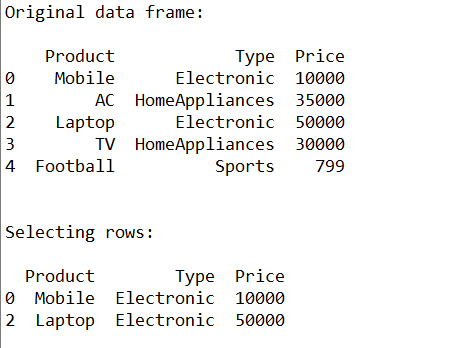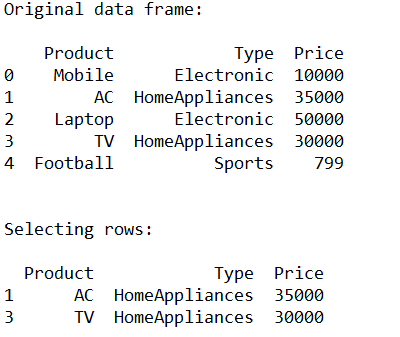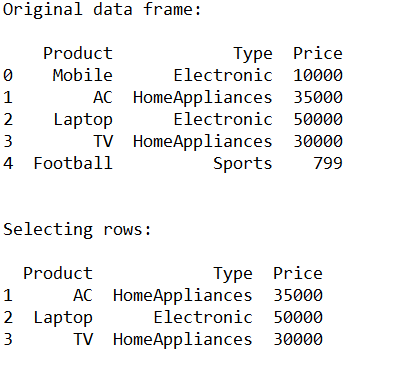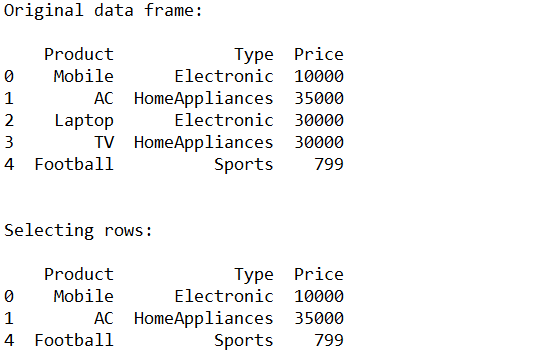# How to Select Rows from Pandas DataFrame?

`pandas.DataFrame.loc` is a function used to select rows from Pandas DataFrame based on the condition provided. In this article, let’s learn to select the rows from Pandas DataFrame based on some conditions.

Syntax: df.loc[df[‘cname’] ‘condition’]

Parameters:
df: represents data frame
cname: represents column name
condition: represents condition on which rows has to be selected

Example 1:

 `# Importing pandas as pd ` `from` `pandas ``import` `DataFrame ` ` `  `# Creating a data frame ` `cart ``=` `{``'Product'``: [``'Mobile'``, ``'AC'``, ``'Laptop'``, ``'TV'``, ``'Football'``], ` `        ``'Type'``: [``'Electronic'``, ``'HomeAppliances'``, ``'Electronic'``,  ` `                 ``'HomeAppliances'``, ``'Sports'``], ` `        ``'Price'``: [``10000``, ``35000``, ``50000``, ``30000``, ``799``] ` `       ``} ` ` `  `df ``=` `DataFrame(cart, columns ``=` `[``'Product'``, ``'Type'``, ``'Price'``]) ` ` `  `# Print original data frame ` `print``(``"Original data frame:\n"``) ` `print``(df) ` ` `  `# Selecting the product of Electronic Type ` `select_prod ``=` `df.loc[df[``'Type'``] ``=``=` `'Electronic'``] ` ` `  `print``(``"\n"``) ` ` `  `# Print selected rows based on the condition ` `print``(``"Selecting rows:\n"``) ` `print` `(select_prod) `

Output:Example 2:

 `# Importing pandas as pd ` `from` `pandas ``import` `DataFrame ` ` `  `# Creating a data frame ` `cart ``=` `{``'Product'``: [``'Mobile'``, ``'AC'``, ``'Laptop'``, ``'TV'``, ``'Football'``], ` `        ``'Type'``: [``'Electronic'``, ``'HomeAppliances'``, ``'Electronic'``, ` `                 ``'HomeAppliances'``, ``'Sports'``], ` `        ``'Price'``: [``10000``, ``35000``, ``50000``, ``30000``, ``799``] ` `       ``} ` ` `  `df ``=` `DataFrame(cart, columns ``=` `[``'Product'``, ``'Type'``, ``'Price'``]) ` ` `  `# Print original data frame ` `print``(``"Original data frame:\n"``) ` `print``(df) ` ` `  `# Selecting the product of HomeAppliances Type ` `select_prod ``=` `df.loc[df[``'Type'``] ``=``=` `'HomeAppliances'``] ` ` `  `print``(``"\n"``) ` ` `  `# Print selected rows based on the condition ` `print``(``"Selecting rows:\n"``) ` `print` `(select_prod) `

Output:Example 3:

 `# Importing pandas as pd ` `from` `pandas ``import` `DataFrame ` ` `  `# Creating a data frame ` `cart ``=` `{``'Product'``: [``'Mobile'``, ``'AC'``, ``'Laptop'``, ``'TV'``, ``'Football'``], ` `        ``'Type'``: [``'Electronic'``, ``'HomeAppliances'``, ``'Electronic'``, ` `                 ``'HomeAppliances'``, ``'Sports'``], ` `        ``'Price'``: [``10000``, ``35000``, ``50000``, ``30000``, ``799``] ` `       ``} ` ` `  `df ``=` `DataFrame(cart, columns ``=` `[``'Product'``, ``'Type'``, ``'Price'``]) ` ` `  `# Print original data frame ` `print``(``"Original data frame:\n"``) ` `print``(df) ` ` `  `# Selecting the product of Price greater  ` `# than or equal to 25000 ` `select_prod ``=` `df.loc[df[``'Price'``] >``=` `25000``] ` ` `  `print``(``"\n"``) ` ` `  `# Print selected rows based on the condition ` `print``(``"Selecting rows:\n"``) ` `print` `(select_prod) `

Output:Example 4:

 `# Importing pandas as pd ` `from` `pandas ``import` `DataFrame ` ` `  `# Creating a data frame ` `cart ``=` `{``'Product'``: [``'Mobile'``, ``'AC'``, ``'Laptop'``, ``'TV'``, ``'Football'``], ` `        ``'Type'``: [``'Electronic'``, ``'HomeAppliances'``, ``'Electronic'``, ` `                 ``'HomeAppliances'``, ``'Sports'``], ` `        ``'Price'``: [``10000``, ``35000``, ``30000``, ``30000``, ``799``] ` `       ``} ` ` `  `df ``=` `DataFrame(cart, columns ``=` `[``'Product'``, ``'Type'``, ``'Price'``]) ` ` `  `# Print original data frame ` `print``(``"Original data frame:\n"``) ` `print``(df) ` ` `  `# Selecting the product of Price not  ` `# equal to 30000 ` `select_prod ``=` `df.loc[df[``'Price'``] !``=` `30000``] ` ` `  `print``(``"\n"``) ` ` `  `# Print selected rows based on the condition ` `print``(``"Selecting rows:\n"``) ` `print` `(select_prod) `

Output:My Personal Notes arrow_drop_upCheck out this Author's contributed articles.

If you like GeeksforGeeks and would like to contribute, you can also write an article using contribute.geeksforgeeks.org or mail your article to contribute@geeksforgeeks.org. See your article appearing on the GeeksforGeeks main page and help other Geeks.

Please Improve this article if you find anything incorrect by clicking on the "Improve Article" button below.

Article Tags :

1

Please write to us at contribute@geeksforgeeks.org to report any issue with the above content.